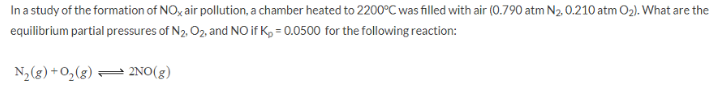# Problem: In a study of the formation of NOx air pollution, a chamber heated to 2200°C was filled with air (0.790 atm N2, 0.210 atm O2). What are the equilibrium partial pressures of N2, O2, and NO if Kp = 0.0500 for the following reaction: N2 (g)+ O2 (g) ⇌ 2NO (g)

###### FREE Expert Solution
85% (150 ratings)###### Problem Details

In a study of the formation of NOx air pollution, a chamber heated to 2200°C was filled with air (0.790 atm N2, 0.210 atm O2). What are the equilibrium partial pressures of N2, O2, and NO if Kp = 0.0500 for the following reaction:

N2 (g)+ O2 (g) ⇌ 2NO (g)